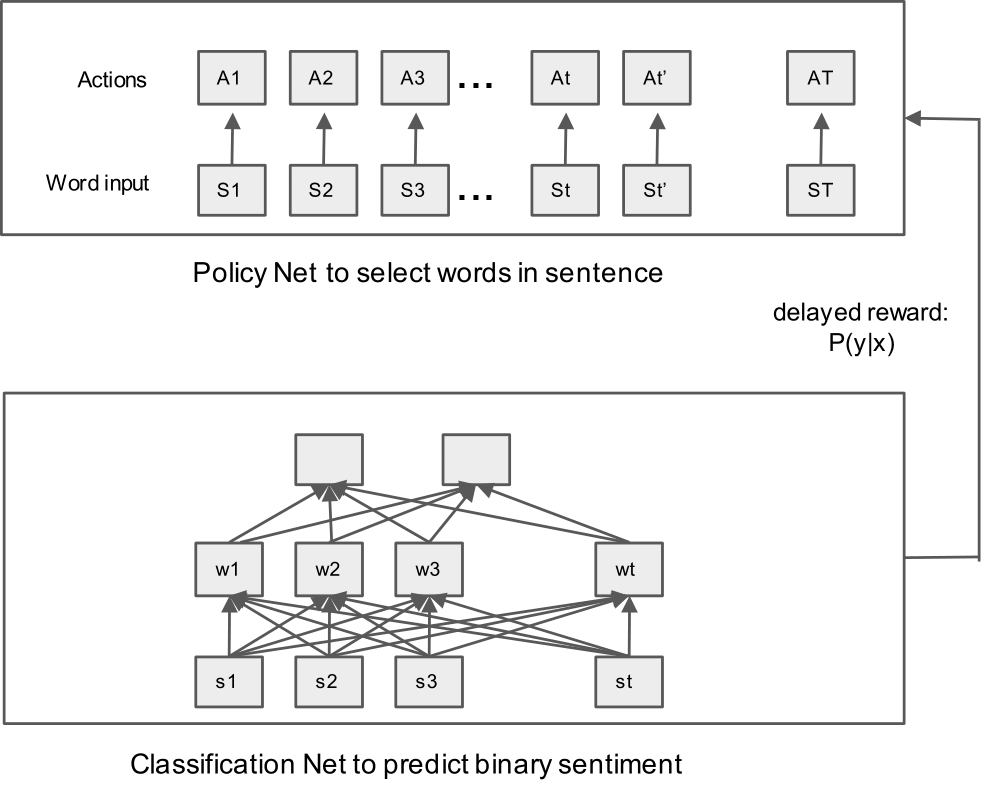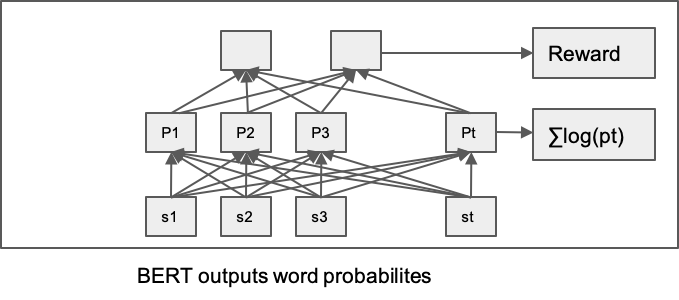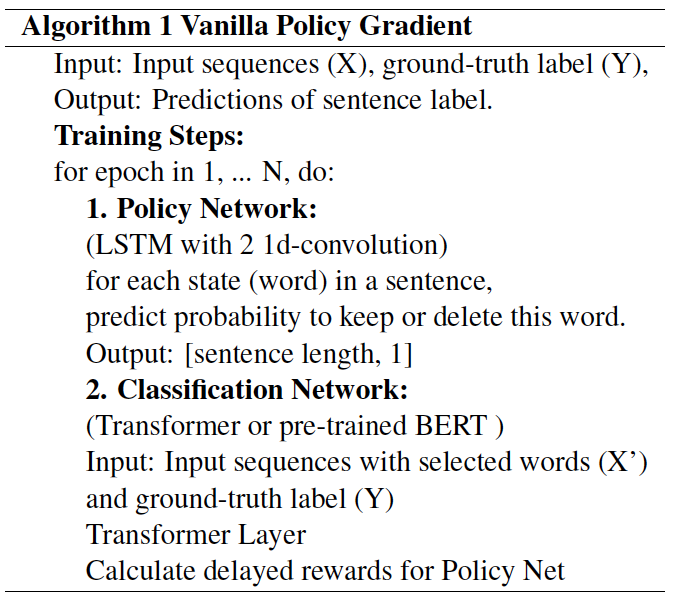Sentiment Analysis Using RL

## Introduction

Representation learning (Bengio et al., 2013) plays an important role in many modern machine learning systems. Natural language processing (NLP) is a hot topic that builds computational algorithms to let computer automatically learn, analyze and represent human language.

In this paper, we propose a novel model that combines reinforcement learning (RL) method and supervised NLP method to predict sentence sentiment. We formulate sentiment-analysis task as a sequential decision process: current decisions (i.e., sentiment) in a sentence affects following decisions (sentiment), which can be naturally addressed by policy gradient method (Sutton et al.,2000). Since we cannot predict the sentiment until reaching end of sentence, we use delayed reward to guide the training process of policy for the problem. We calculate the reward based on the supervised learning with selected inputs from RL method.

To summarize, the main contributions and novelties are as follows:

• We propose an RL method to discover useful and meaningful words in a sentence to predict sentiment.
• The performance is better or comparable to current baselines using supervised learning algorithm.

## Methodology

Our goal of this project is to combine RL method for sentiment analysis besides supervised learning. We proposed two structures to improve the prediction of sentence sentiment.

### Policy + Classification Network

The overall process is shown in the figure. In Policy Net, it uses simple LSTM to generate state values and sample action at each word. It keeps sampling until the end of a sentence, which will produce a sequence of actions for the sentence. Then we translate the actions into an input word sequence for supervised learning algorithm. For the Policy Network, we use LSTM and for the Classification Network, we adopt transformer and pre-trained BERT (bert-base-uncased) and then calculate delayed reward to Policy Network. Given the delayed reward after the full sentence, the process can be naturally addressed by policy gradient method (Konda and Tsitsiklis, 2000), actor-critic method and proximal policy optimization (Schulman et al., 2017).#### Policy Network

The policy network is based on well-trained LSTM using pre-trained BERT embeddings to produce state values ($s_t$) and action sequence (a_t). It adopts the policy $\pi(a_t | s_t)$ and a delayed reward to guide the policy optimization. At each state (i.e., word) it samples an action with probability. In specific, the binary action space can be explained as {remain, delete}, i.e., whether keep or delete the word in the sentence to predict sentence sentiment. Then the policy is defined as:Based on the action sequence, we then pass the selected words to Classification Network to compute probability of the binary sentence sentiment. For policy optimization, we apply three different methods with different objective functions respectively.#### Classification Network

Based on the action sequence, we then pass the selected words to Classification Network to compute $P(y|X)$, where y is the binary sentence sentiment. We adopt LSTM, transformer and pre-trained BERT as model structure and binary cross entropy as loss function:#### Training Details

The entire training process consists of following steps: policy network heavily relies on well-tuned LSTM model, and classification network is then jointly trained together with policy network. The sudo algorithms are listed in the following section.

### Word Sentiment Network

We propose a second algorithm that combines RL and supervised learning method for sentiment analysis. For each state (i.e., word) in a sentence, we adopt pre-trained BERT to output two probabilities of positive sentiment, following forward sentence order and backward sentence order respectively. We then develop several loss functions as follows:

• Use only the forward probabilities, with reward defined as:• Use two loss terms with same definition as previous one, and then adding them up;
• Add the forward and backward log probabilities together and use it in PPO ratio.where two probabilities are predicted probability given forward or backward sentence order inputs.

### Algorithms

We consider two structures as discussed above. For the policy and classification networks, the algorithms are listed as follows with respect to different RL methods:## Experiments

### Dataset and Baselines

We evaluated our models on SST (Stanford Sentiment Treebank, a public sentiment analysis dataset with five classes (Socher et al., 2013)) dataset for sentiment classification.

Models Accuracy AUC
LSTM 0.7403
Transformer 0.7981
Transformer + VPG 0.7688
Transformer + AC 0.7946
Transformer + PPO 0.8045

## Conclusion

This project presented models that combine reinforcement learning and supervised learning methods for language sentiment analysis. For the model that involves policy network and classification network, we find adding reinforcement learning method can improve the performance from transformer model and produce comparable results on pre-trained BERT model.

## Reference

Yoshua Bengio, Aaron Courville, and Pascal Vincent.2013. Representation learning: A review and new perspectives.IEEE transactions on pattern analysis and machine intelligence, 35(8):1798 - 1828.

Vijay R Konda and John N Tsitsiklis. 2000. Actor-critic algorithms. InAdvances in neural information processing systems, pages 1008 - 1014.

John Schulman, Filip Wolski, Prafulla Dhariwal,Alec Radford, and Oleg Klimov. 2017.Proximal policy optimization algorithms.arXiv preprint arXiv:1707.06347.

Richard Socher, Alex Perelygin, Jean Wu, JasonChuang, Christopher D Manning, Andrew Ng, andChristopher Potts. 2013.Recursive deep models for semantic compositionality over a sentiment tree-bank. InProceedings of the 2013 conference on empirical methods in natural language processing, pages 1631 - 1642.

Richard S Sutton, David A McAllester, Satinder PSingh, and Yishay Mansour. 2000. Policy gradient methods for reinforcement learning with function approximation. InAdvances in neural information processing systems, pages 1057 - 1063.